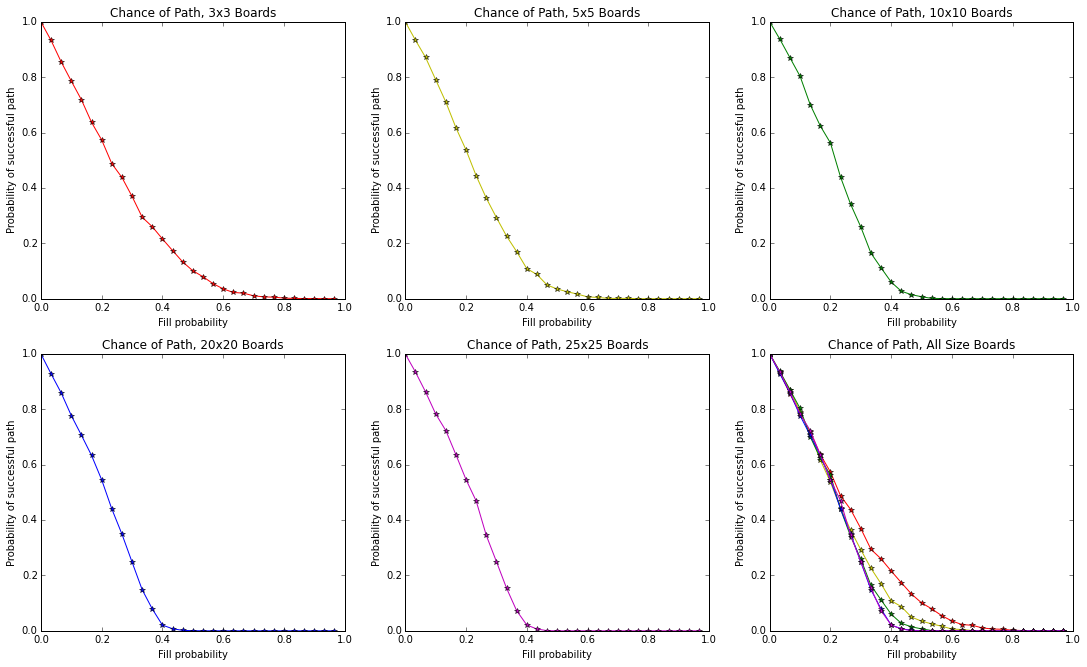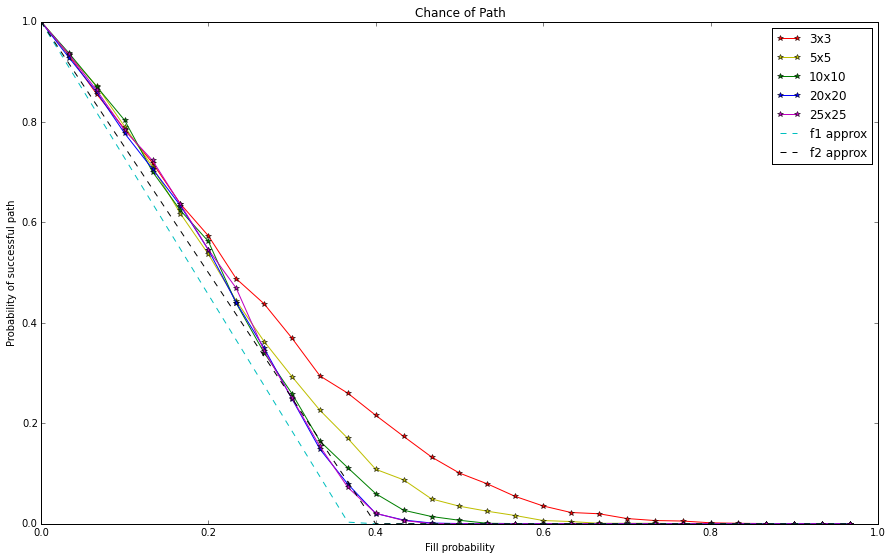## Let's make a maze!¶

In :
%matplotlib inline
import math, numpy as np, matplotlib.pyplot as plt

class Maze():
def __init__(self,size=10,fill=0.1):
self.size = size
self.board = np.zeros([size,size])
for row in self.board:
for i,item in enumerate(row):
row[i] = np.random.random() < fill #1 designates filled, 0 is empty
def view(self):
print(self.board)
def find_path(self,start=(0,0),end=(-1,-1)):
start_i,start_j = start % self.size, start % self.size
end_i,end_j = end % self.size, end % self.size
result = self._recurse_find_path(start_i,start_j,end_i,end_j) if self.board[start_i][start_j] == 0 and self.board[end_i][end_j] == 0 else False
self._remove_7s()
return result
def _recurse_find_path(self,i,j,end_i,end_j):
self.board[i][j] = 3 #3 designates path
if i == end_i and j == end_j:
return True
options = [(i,j+1),(i+1,j),(i-1,j),(i,j-1)]
for x,y in options:
if self._ij_legal(x,y) and self.board[x][y] == 0:
if self._recurse_find_path(x,y,end_i,end_j):
return True
self.board[i][j] = 7 #7 designates visited
return False
def _ij_legal(self,i,j):
return i == int(i) and j == int(j) and i >=0 and j >=0 and i < self.size and j < self.size
def _remove_7s(self):
for row in self.board:
for i,item in enumerate(row):
if item == 7:
row[i] = 0
def clear_path(self):
for row in self.board:
for i,item in enumerate(row):
if item != 1:
row[i] = 0


## Okay!¶

Use Maze(arg1,arg2) to make a maze of size arg1xarg1, where each square has fill probability arg2.

In :
x = Maze(8,0.2)
x.view()

[[ 0.  0.  0.  0.  0.  1.  0.  0.]
[ 0.  0.  0.  0.  0.  1.  0.  1.]
[ 0.  0.  0.  0.  0.  0.  0.  0.]
[ 0.  0.  1.  0.  0.  0.  0.  0.]
[ 0.  0.  0.  0.  0.  0.  1.  1.]
[ 0.  0.  1.  0.  1.  1.  0.  0.]
[ 1.  0.  1.  0.  0.  0.  0.  0.]
[ 1.  0.  1.  0.  0.  0.  1.  1.]]


## Path-finding¶

The method find_path() will attempt to find a path through the maze and return success or failure. If successful, the path will be populated with 3s.

In :
print(x.find_path())
x.view()

False
[[ 0.  0.  0.  0.  0.  1.  0.  0.]
[ 0.  0.  0.  0.  0.  1.  0.  1.]
[ 0.  0.  0.  0.  0.  0.  0.  0.]
[ 0.  0.  1.  0.  0.  0.  0.  0.]
[ 0.  0.  0.  0.  0.  0.  1.  1.]
[ 0.  0.  1.  0.  1.  1.  0.  0.]
[ 1.  0.  1.  0.  0.  0.  0.  0.]
[ 1.  0.  1.  0.  0.  0.  1.  1.]]

In :
x.clear_path()
x.view()

[[ 0.  0.  0.  0.  0.  1.  0.  0.]
[ 0.  0.  0.  0.  0.  1.  0.  1.]
[ 0.  0.  0.  0.  0.  0.  0.  0.]
[ 0.  0.  1.  0.  0.  0.  0.  0.]
[ 0.  0.  0.  0.  0.  0.  1.  1.]
[ 0.  0.  1.  0.  1.  1.  0.  0.]
[ 1.  0.  1.  0.  0.  0.  0.  0.]
[ 1.  0.  1.  0.  0.  0.  1.  1.]]


Use find_path(start_coord,end_coord) to find a path between specific coordinates (default is top left corner to bottom right corner).

In :
print(x.find_path((2,2),(-2,-2)))
x.view()

True
[[ 0.  0.  0.  0.  0.  1.  0.  0.]
[ 0.  0.  0.  0.  0.  1.  0.  1.]
[ 0.  0.  3.  3.  3.  3.  3.  3.]
[ 0.  0.  1.  3.  3.  3.  3.  3.]
[ 0.  0.  0.  3.  3.  3.  1.  1.]
[ 0.  0.  1.  3.  1.  1.  0.  0.]
[ 1.  0.  1.  3.  3.  3.  3.  0.]
[ 1.  0.  1.  0.  0.  0.  1.  1.]]


Careful! The path will persist on the board unless you remove it with clear_path.

In :
x.clear_path()
x.view()
print(x.find_path((5,5),(6,7)))
x.view()

[[ 0.  0.  0.  0.  0.  1.  0.  0.]
[ 0.  0.  0.  0.  0.  1.  0.  1.]
[ 0.  0.  0.  0.  0.  0.  0.  0.]
[ 0.  0.  1.  0.  0.  0.  0.  0.]
[ 0.  0.  0.  0.  0.  0.  1.  1.]
[ 0.  0.  1.  0.  1.  1.  0.  0.]
[ 1.  0.  1.  0.  0.  0.  0.  0.]
[ 1.  0.  1.  0.  0.  0.  1.  1.]]
False
[[ 0.  0.  0.  0.  0.  1.  0.  0.]
[ 0.  0.  0.  0.  0.  1.  0.  1.]
[ 0.  0.  0.  0.  0.  0.  0.  0.]
[ 0.  0.  1.  0.  0.  0.  0.  0.]
[ 0.  0.  0.  0.  0.  0.  1.  1.]
[ 0.  0.  1.  0.  1.  1.  0.  0.]
[ 1.  0.  1.  0.  0.  0.  0.  0.]
[ 1.  0.  1.  0.  0.  0.  1.  1.]]


## Path Statistics¶

How likely are you to find a path from the top left corner to the bottom right corner? One can conduct an experiment and watch how path success varies with fill probability and board size.

In :
from __future__ import division
sizes = [3,5,10,20,25]
splits = 30
trials = 2000
averages = []
colors = ['r-*','y-*','g-*','b-*','m-*']
fig, axes = plt.subplots(figsize=(15,9))
fig.tight_layout()
for k,size in enumerate(sizes):
averages.append(*splits)
for j,fill in [(i,i/splits) for i in range(0,splits)]:
for _ in range(trials):
x = Maze(size,fill)
if x.find_path():
averages[k][j] += 1
averages[k] = [i/trials for i in averages[k]]
plt.subplot(231+k)
plt.xlabel("Fill probability")
plt.ylabel("Probability of successful path")
plt.title("Chance of Path, " + str(sizes[k]) + "x" + str(sizes[k]) + " Boards")
plt.plot([i/splits for i in range(0,splits)],averages[k],colors[k % len(colors)])
for k in range(len(averages)):
plt.subplot(236)
plt.xlabel("Fill probability")
plt.ylabel("Probability of successful path")
plt.title("Chance of Path, All Size Boards")
plt.plot([i/splits for i in range(0,splits)],averages[k],colors[k % len(colors)])## Approximations¶

Here I've tried to approximate the plots shown above with different curves. It seems like the path probability generally varies linearly with fill probability. When the path chance is near zero, smaller-size boards have better luck.

In :
def f1(x):
y = 1 - x/(splits*math.exp(-1)) #e^-1 is roughly 0.36787944117144233
return max(y,0)
def f2(x):
y = 1 - x/(splits*0.4)
return max(y,0)

fig = plt.figure(figsize=(15,9))
for k in range(len(averages)):
plt.plot([i/splits for i in range(0,splits)],averages[k],colors[k % len(colors)])
plt.plot([i/splits for i in range(0,splits)],[f1(x) for x in range(0,splits)], 'c--')
plt.plot([i/splits for i in range(0,splits)],[f2(x) for x in range(0,splits)], 'k--')
plt.xlabel("Fill probability")
plt.ylabel("Probability of successful path")
plt.title("Chance of Path")
legend = [str(size) + "x" + str(size) for size in sizes]
legend.append("f1 approx")
legend.append("f2 approx")
plt.legend(legend)

Out:
<matplotlib.legend.Legend at 0xb899940>## Cool!¶

Future work involves probability theory to predict the true chance of a path given the board size. We can validate this theoretical work with the simulations above. For now, we can make and play with the mazes. Thanks for playing!

In :
x = Maze(10,0.2)
x.view()
print(x.find_path())
x.view()

[[ 0.  0.  0.  1.  0.  0.  0.  1.  1.  0.]
[ 0.  0.  0.  1.  1.  0.  0.  0.  0.  1.]
[ 0.  0.  0.  0.  1.  0.  0.  0.  0.  0.]
[ 1.  0.  0.  0.  0.  0.  0.  1.  0.  0.]
[ 0.  0.  0.  0.  0.  1.  0.  0.  0.  0.]
[ 1.  1.  0.  0.  1.  0.  0.  1.  0.  0.]
[ 0.  1.  1.  0.  0.  0.  0.  0.  0.  0.]
[ 0.  0.  0.  1.  0.  0.  0.  1.  1.  1.]
[ 0.  0.  0.  1.  0.  0.  0.  0.  0.  1.]
[ 0.  0.  0.  0.  0.  0.  1.  0.  0.  0.]]
True
[[ 3.  3.  3.  1.  0.  0.  0.  1.  1.  0.]
[ 0.  0.  3.  1.  1.  0.  0.  0.  0.  1.]
[ 0.  0.  3.  3.  1.  0.  0.  0.  0.  0.]
[ 1.  0.  0.  3.  3.  3.  3.  1.  0.  0.]
[ 0.  0.  0.  0.  0.  1.  3.  3.  3.  3.]
[ 1.  1.  0.  0.  1.  0.  0.  1.  0.  3.]
[ 0.  1.  1.  0.  0.  0.  3.  3.  3.  3.]
[ 0.  0.  0.  1.  0.  0.  3.  1.  1.  1.]
[ 0.  0.  0.  1.  0.  0.  3.  3.  3.  1.]
[ 0.  0.  0.  0.  0.  0.  1.  0.  3.  3.]]

In [ ]: